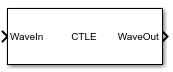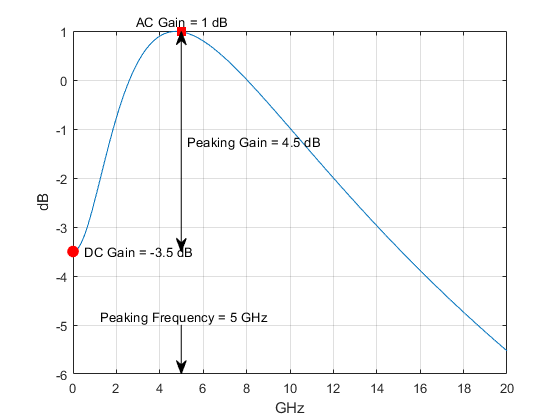Main Content

# CTLE

Models continuous time linear equalizer (CTLE)

• Library:
• SerDes Toolbox / Datapath Blocks

•## Description

The CTLE block applies a linear peaking filter to equalize the frequency response of a sample-by-sample input signal. The equalization process reduces distortions resulting from lossy channels. The filter is a real one-zero two-pole (1z/2p) filter, unless you define the gain-pole-zero (GPZ) matrix.

## Ports

### Input

expand all

Input baseband signal. The input signal can be a sample-by-sample signal specified as a scalar, or an impulse response vector signal.

Data Types: `double`

### Output

expand all

Equalized CTLE output waveform. If the input signal is a sample-by-sample signal specified as a scalar, then the output is also scalar. If the input signal is an impulse response vector signal, then the output is also a vector.

Data Types: `double`

## Parameters

expand all

CTLE operating mode:

• `Off`CTLE is bypassed and the input waveform remains unchanged.

• `Fixed`CTLE applies the CTLE transfer function as specified by Configuration select to the input waveform.

• `Adapt` — If the input signal is an impulse response vector or a waveform vector, then the Init subsystem calls to the CTLE System object™. The CTLE System object determines the CTLE transfer function to maximize the SNR and applies the transfer function to the input waveform for time domain simulation. This optimized transfer function is used by the CTLE for entire time domain simulation. For more information about the Init subsystem, see Statistical Analysis in SerDes Systems

If the input signal is a sample-by-sample scalar, then the CTLE operates in the `Fixed` mode.

#### Programmatic Use

• Use `get_param(gcb,'Mode')` to view the current CTLE Mode.

• Use `set_param(gcb,'Mode',value)` to set CTLE to a specific Mode.

Select which transfer function configuration to apply in CTLE fixed mode, specified as a real integer scalar. Depending on the Specification, Configuration select specifies which gain coefficient is applied to the filter transfer function.

For example, setting Configuration select to n and Specification to ```'DC Gain and Peaking Gain'``` selects the (n+1)-th element in the DC gain (dB) and Peaking gain (dB) vectors to be applied to the filter transfer function.

If CTLE Mode is set to `Adapt` and the input is an impulse response vector or a waveform vector, Configuration select is automatically calculated to determine the best eye height opening. To view the value of the Configuration select parameter, choose Add Plots > Report in the SerDes Designer app.

#### Programmatic Use

• Use `get_param(gcb,'ConfigSelect')` to view the current value of Configuration Select.

• Use `set_param(gcb,'ConfigSelect',value)` to set Configuration Select to a specific value.

Data Types: `double`

Defines which inputs will be used for the CTLE transfer function family. There are five inputs which can be used to define the CTLE transfer function family: DC gain (dB), Peaking gain (dB), AC gain (dB), Peaking frequency (Hz), and Gain pole zero matrix.

You can define the CTLE response from any two of the three gains and peaking frequency or you can define the GPZ matrix for the CTLE.• Select `'DC Gain and Peaking Gain'` to specify CTLE response from DC gain (dB), Peaking gain (dB), and Peaking frequency (Hz).

• Select `'DC Gain and AC Gain'` to specify CTLE response is from DC gain (dB), AC gain (dB), and Peaking frequency (Hz).

• Select `'AC Gain and Peaking Gain'` to specify CTLE response from AC gain (dB), Peaking gain (dB), and Peaking frequency (Hz).

• Select `'GPZ Matrix'` to specify CTLE response is from Gain pole zero matrix.

#### Programmatic Use

• Use `get_param(gcb,'Specification')` to view the current CTLE Specification.

• Use `set_param(gcb,'Specification',value)` to set CTLE to a specific Specification.

Data Types: `char`

Gain at zero frequency for the CTLE transfer function, specified as a scalar or a vector in dB. If specified as a scalar, it is converted to match the length of Peaking gain (dB), AC gain (dB), and Peaking frequency (Hz) by scalar expansion. If specified as a vector, the vector length must be the same as the vectors in Peaking gain (dB), AC gain (dB), and Peaking frequency (Hz).

#### Dependencies

This parameter is only available when Specification is set to `'DC Gain and Peaking Gain'` or ```'DC Gain and AC Gain'```.

#### Programmatic Use

• Use `get_param(gcb,'DCGain')` to view the current value of DC gain (dB).

• Use `set_param(gcb,'DCGain',value)` to set DC gain (dB) to a specific value.

Data Types: `double`

Peaking gain, specified as a scalar or vector in dB. Peaking gain (dB) is the difference between AC gain (dB) and DC gain (dB) for the CTLE transfer function. If specified as a scalar, it is converted to match the length of DC gain (dB), AC gain (dB), and Peaking frequency (Hz) by scalar expansion. If specified as a vector, the vector length must be the same as the vectors in DC gain (dB), AC gain (dB), and Peaking frequency (Hz).

#### Dependencies

This parameter is only available when Specification is set to `'DC Gain and Peaking Gain'` or ```'AC Gain and Peaking Gain'```.

#### Programmatic Use

• Use `get_param(gcb,'PeakingGain')` to view the current value of Peaking gain (dB).

• Use `set_param(gcb,'PeakingGain',value)` to set Peaking gain (dB) to a specific value.

Data Types: `double`

Gain at the peaking frequency for the CTLE transfer function, specified as a scalar or vector in dB. If specified as a scalar, it is converted to match the length of DC gain (dB), Peaking gain (dB), and Peaking frequency (Hz) by scalar expansion. If specified as a vector, the vector length be the same as the vectors in DC gain (dB), Peaking gain (dB), and Peaking frequency (Hz).

#### Dependencies

This parameter is only available when Specification is set to `'DC Gain and AC Gain'` or ```'AC Gain and Peaking Gain'```.

#### Programmatic Use

• Use `get_param(gcb,'ACGain')` to view the current value of AC gain (dB).

• Use `set_param(gcb,'ACGain',value)` to set AC gain (dB) to a specific value.

Data Types: `double`

Approximate frequency at which CTLE transfer function peaks in magnitude, specified as a scalar or a vector in GHz. If specified as a scalar, it is converted to match the length of DC gain (dB), AC gain (dB), and Peaking gain (dB) by scalar expansion. If specified as a vector, the vector length must be the same as the vectors in DC gain (dB), AC gain (dB), and Peaking gain (dB).

#### Dependencies

This parameter is not available when Specification is set to `'GPZ Matrix'` .

#### Programmatic Use

• Use `get_param(gcb,'PeakingFrequency')` to view the current value of Peaking frequency (Hz).

• Use `set_param(gcb,'PeakingFrequency',value)` to set Peaking frequency (Hz) to a specific value.

Data Types: `double`

Gain pole zero, specified as a matrix. Gain pole zero matrix explicitly defines the family of CTLE transfer functions by specifying the DC gain (dB) (dB) in the first column and then poles and zeros in alternating columns. The poles and zeros are specified in Hz. Additional rows in the matrix define additional configurations, which can be selected using the Configuration Select parameter.

No repeated poles or zeros are allowed. Complex poles or zeros must have conjugates. The number of poles must be greater than number of zeros for system stability. Poles and zeros of 0 Hz is ignored and can be used to zero-pad the matrix.

Example: To create a gain pole zero matrix with three poles and two zeroes, input the matrix as follows: [G, P1, Z1, P2, Z2, P3].

#### Dependencies

This parameter is only available when Specification is set to `'GPZ Matrix'`.

#### Programmatic Use

• Use `get_param(gcb,'GPZ')` to view the current value of Gain pole zero matrix.

• Use `set_param(gcb,'GPZ',value)` to set Gain pole zero matrix to a specific value.

Data Types: `double`
Complex Number Support: Yes

Click to plot the CTLE response. The resulting plot shows CTLE transfer function family curves for different CTLE configurations.

IBIS-AMI parameters

Select to include Mode as a parameter in the IBIS-AMI file. If you deselect Mode, it is removed from the AMI files, effectively hard-coding Mode to its current value.

Select to include Configuration select as a parameter in the IBIS-AMI file. If you deselect Configuration select, it is removed from the AMI files, effectively hard-coding Configuration select to its current value.

## See Also

Introduced in R2019a

## SupportGet trial now